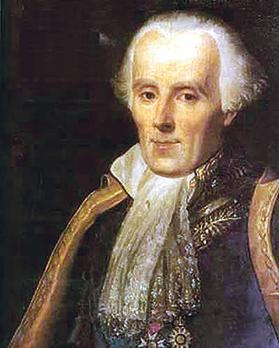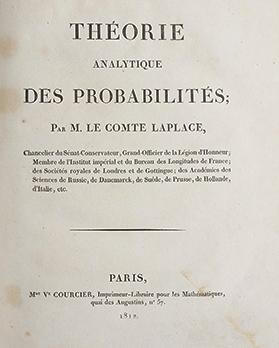Pierre-Simon Laplace. Photo: https://en.wikiquote.org/wiki/Pierre-Simon_Laplace.

The theory of probability had its origins in games of chance and gambling. Probability originated from a gambler’s dispute in 1654 concerning the division of a stake between two players whose game was interrupted before its close. The problem was proposed by a well-to-do gambler, the Chevalier de Mere to prominent mathematicians including Blaise Pascal who shared his thoughts with Pierre de Fermat.

The correspondence which ensued between the two mathematicians was fundamental in the development of modern concepts of probability. From a mathematical point of view, games of chance are experiments generating various types of aleatory events, the probability of which can be calculated by using the properties of probability on a finite space of events.

The work of Christiaan Huygens, in 1657, is a systematic treatise on probability and deals with games of chance and the problem of points – what today is known as expectation values.The book Théorie Analytique des Probabilités by Pierre-Simon Laplace. PHOTO: https://www.sophiararebooks.com/pages/books/4159/pierre-simon-laplace-marquis-de/theorie-analytique-des-probabilites-paris-courcier-1812-with-supplement-a-la-theorie-analytique

The first attempt at some mathematical rigour is credited to Pierre-Simon Laplace.

In 1812, Laplace issued his Théorie Analytique des Probabilités in which he gave the classical definition of the probability of a discrete event, which is the proportion of the number of favourable outcomes to the total finite number of all possible outcomes, given that all outcomes are equally likely.

The methods used to compute these probabilities were mainly combinatorial. Till the end of the 19th century, Laplace’s contribution remained the most influential of mathematical probability theory.

An extension of Laplace’s classical definition incorporated the probabilities of events with infinite outcomes, where the notion of equal likelihood of events was fundamental in its development.

According to this extension, the sample space could be any region with a well-defined measure (length, area, volume, etc.), where the number of points is uncountable. Many problems of geometric probability were solved using this extension.

The mathematical theory of probability as we know it today was developed by Andrey Kolmogorov.

In 1933, Kolmogorov issued his Foundations of the Theory of Probability, where he combined the notion of sample space and measure theory to axiomatise probability.

In this measure-theoretic development, random events are represented by sets and the probability of an event is just a normed measure defined on these sets. Besides becoming the undisputed axiomatic basis for modern probability theory, Kolmogorov’s work provided a logically consistent foundation for probability theory and joined it to the mainstream of modern mathematics.

## Sound bites

• From the international scene: In 1972, Nelder and Wedderburn introduced the concept of Generalised Linear Models (GLM), which extended Regression Models to accommodate any distribution in the exponential family of distributions. A fundamental assumption of GLMs is that the responses are independent and that these models are not appropriate for longitudinal or nested data. This limitation paved way to two different methodologies adequate for dependence and correlation between the observations. In 1986, Liang and Zeger introduced the concept of Generalised Estimating Equations (GEE).  GEE models drop the assumption of independence and accommodate highly correlated data by specifying a structure for the working correlation matrix. One of the limitations of GEE models is that they do not accommodate data that has a nested hierarchical structure.  In 1992, Bryk and Raudenbush introduced the concept of Multilevel models, also known as hierarchical linear models. These models differ from GLM and GEE models in the fact that the equations defining the hierarchical linear models contain an error term at each hierarchy, and this makes it possible to estimate the variance at each level of nesting. Moreover, these models accommodate both fixed and random effects.

• From the local scene: The author of this page (Liberato Camilleri) applied these statistical models in several research fields including social well-being, education, insurance and mortality through the following publications:

‘Social, emotional and behaviour difficulties in Maltese schools: A multilevel model’. Journal of Research in Educational Sciences, Volume 2, Issue 1, Number 3, p.3-15.

‘Using multilevel random coefficient models to assess students’ spelling abilities’. Proceedings of the 24th ESM Conference, p. 449-454.

‘Fitting generalised linear models to car claims data. Proceedings of the 5th International Conference on Financial and Actuarial Mathematics, p. 6-17.

‘Modelling mortality rates using GEE models’. Proceedings of the 4th Stochastic Modelling Techniques and Data Analysis International Conference, p.69-80.

For more science news, listen to Radio Mocha on Radju Malta 2 every Saturday at 11.05am.

## Did you know?

• The probability of winning the full prize of the Super 5 lottery is approximately nine times the probability of dying in a plane crash.

• The probability of being struck by a lightning at some point during your lifetime is around 22 times the probability of being attacked by a shark.

• The probability of dying from a local meteorite, asteroid or comet impact is almost the same as the probability of scoring six, on the toss of a fair die, eight times in succession.

• The probability of being born with 11 fingers or toes is around 23 times the probability of winning an Oscar.

• The probability of finding a pearl in an oyster is approximately 775 times the probability of getting bombed by a terrorist.

• The probability of being involved in a car accident is around 24 times the probability of fatally slipping in bath or shower.

For more trivia, go to www.um.edu.mt/think

Independent journalism costs money. Support Times of Malta for the price of a coffee.

Support Us